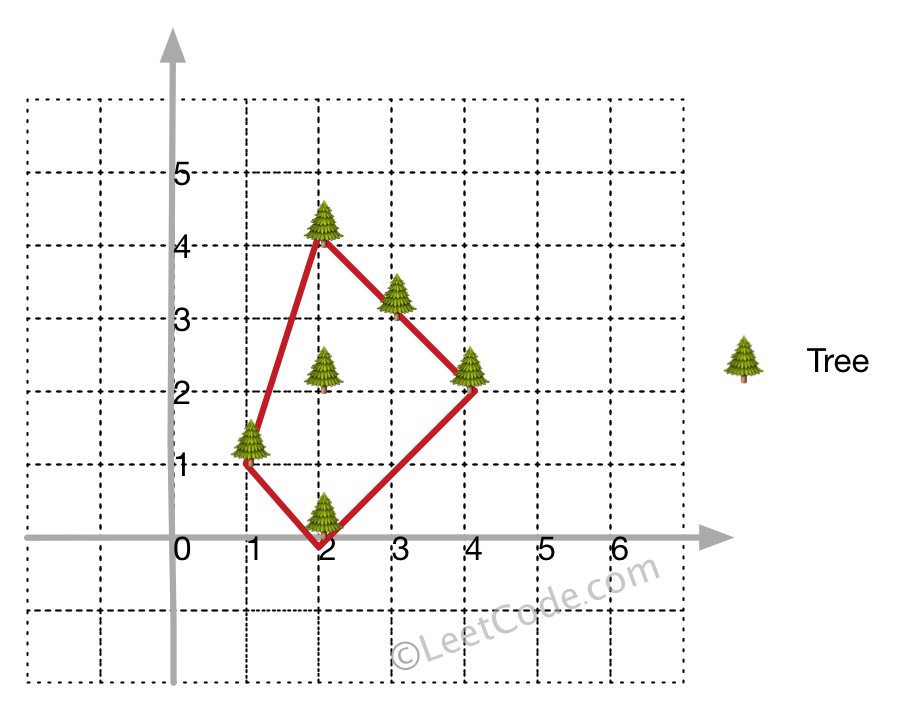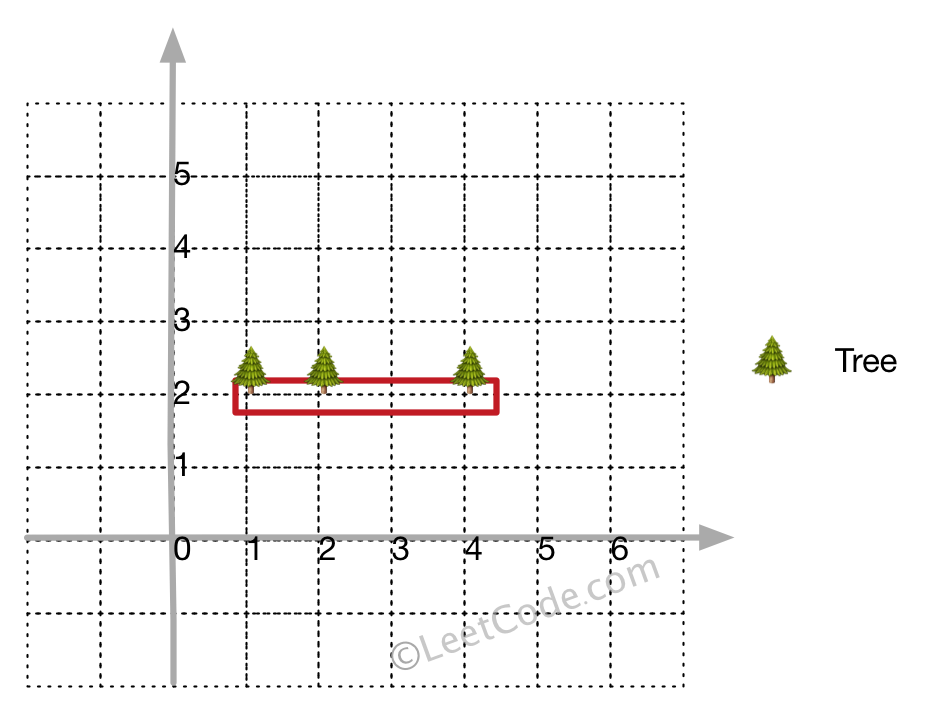# 587. Erect the Fence (Hard)

https://leetcode.com/problems/erect-the-fence/

There are some trees, where each tree is represented by (x,y) coordinate in a two-dimensional garden. Your job is to fence the entire garden using the minimum length of rope as it is expensive. The garden is well fenced only if all the trees are enclosed. Your task is to help find the coordinates of trees which are exactly located on the fence perimeter.

Example 1:

```Input: [[1,1],[2,2],[2,0],[2,4],[3,3],[4,2]]
Output: [[1,1],[2,0],[4,2],[3,3],[2,4]]
Explanation:```

Example 2:

```Input: [[1,2],[2,2],[4,2]]
Output: [[1,2],[2,2],[4,2]]
Explanation:Even you only have trees in a line, you need to use rope to enclose them.
```

Note:

1. All trees should be enclosed together. You cannot cut the rope to enclose trees that will separate them in more than one group.
2. All input integers will range from 0 to 100.
3. The garden has at least one tree.
4. All coordinates are distinct.
5. Input points have NO order. No order required for output.

## Solutions

``````class Solution {
// this problem is too complicated, skip it. Go through this problem with example
// int[][] points = new int[][]{{0, 0}, {1, 1}, {2, 2}, {1, 2}, {2, 4}, {0, 2}, {0, 3}, {-1, 1}, {-2, 2}};

class Point {
int x;
int y;
public Point(int x, int y) {
this.x = x;
this.y = y;
}
}

public int[][] outerTrees(int[][] xpoints) {
Point[] points = new Point[xpoints.length];
for (int i = 0; i < xpoints.length; i++) {
points[i] = new Point(xpoints[i], xpoints[i]);
}

int size = points.length;

// if less than 3 points, all included as answer
if (size <= 3) {
return xpoints;
}

List<Point> ans = new ArrayList<>();

// minXY will serve as the original point on the coordinate
int minXY = 0;

for (int i = 1; i < size; ++i) {
// if (points[i].y > points[minXY].y, minXY stays the same

if (points[i].y < points[minXY].y) {
minXY = i;
}

// minXY point must be the most left bottom one
if (points[i].y == points[minXY].y && points[i].x < points[minXY].x) {
minXY = i;
}
}

// swap points at index 0 and minXY to place minXY at the head
if (minXY != 0) {
Point t = points;
points = points[minXY];
points[minXY] = t;
}

// the original point must be included

// sort the points according to the angle between positive x axis and the vector
// linked from original point to current point by ascending order
Arrays.sort(points, 1, size, new PointsComparator(points.x, points.y));

// Here we talk about the points, loosely refer to the vectors starting from original point
// minXY to them

int idx = size - 1;

// Find out points that parallel to the leftmost points
while (idx >= 0 && crossProduct(points, points[size-1], points[idx]) == 0) {
idx--;
}

// In terms of sorting rules, the point[size-1] consists of the leftmost vector on axis
// and longest when compared to its parallel points.

// Others parallel to point[size-1] but closer to original point will be automatically covered
// when the fence is built up. So it is not necessary to go through these points

int i = idx + 1;
int j = size - 1;

// swap parallel points i and j, the further one move to front
while (i < j) {
Point t = points[i];
points[i] = points[j];
points[j] = t;

i++;
j--;
}

// now the points are organized more like a fence, say the right most points are
// visited from shorter to longer, and the left most points are visited from longer to
// shorter so that the iteration could end up at the original point.

// If not reverse the parallel leftmost points, the closer points will be ignored.
// For nodes internal fence it is allowed, but for points on the boundary of fence,
// all should be kept.

// points is the rightmost vector, but closest when it has parallel points.
// Apparently, we should start from the left most and closest point

int k = 2;
while (k < size) {
while (ans.size() >= 2) {
// k is increasing, however, the order is from right axis to left axis, happened to be opposite
// We can imaging that we are building fence from right axis to left axis.

// These to be added points must be on the left of the vector that formed with newly added two points.
// So that the every points will be enclosed by the fence.
int c = crossProduct(ans.get(ans.size() - 2), ans.get(ans.size() - 1), points[k]);
if (c >= 0) {
break;
}

// if points[k] can not be enclosed.
if (c < 0) {
ans.remove(ans.size() - 1);
}
}

k++;
}

int[][] xans = new int[ans.size()];
for (int t = 0; t < ans.size(); t++) {
xans[t] = ans.get(t).x;
xans[t] = ans.get(t).y;
}

return xans;
}

// Horizontally, we have three situations.
// 1. oa on the left of ob if production < 0,
// 2. ob on the left of oa if production > 0
// 3. and parallel if production = 0
private int crossProduct(Point org, Point a, Point b) {
// cross production of vector origin->a oa and origin->b ob
return (a.x - org.x) * (b.y - org.y) - (a.y - org.y) * (b.x - org.x);
}

private class PointsComparator implements Comparator<Point> {
// original point of the plot
private Point op;

public PointsComparator(int x, int y) {
op = new Point(x, y);
}

public int compare(Point a, Point b) {
int c = crossProduct(op, a, b);

// Horizontally, right side point moves to head of point list

// Horizontally, a on right of b
if (c > 0) {
return -1;
}

// Horizontally, b on right of a
if (c < 0) {
return 1;
}

// parallel reaches here

// distance to the origin point
int disa = (op.x - a.x) * (op.x - a.x) + (op.y - a.y) * (op.y - a.y);
int disb = (op.x - b.x) * (op.x - b.x) + (op.y - b.y) * (op.y - b.y);

// further points moves to tail of the points list
return disa - disb;
}
}
}
``````